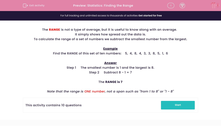# Statistics: Finding the Range

In this worksheet, students calculate the range of a given set of data.Key stage:  KS 2

Curriculum topic:   Maths and Numerical Reasoning

Curriculum subtopic:   Mean, Median, Mode and Range

Difficulty level:#### Worksheet Overview

The RANGE is not a type of average, but it is useful to know along with an average.

It simply shows how spread out the data is.

To calculate the range of a set of numbers we subtract the smallest number from the largest.

Example

Find the RANGE of this set of ten numbers:    5,  4,  8,  4,  3,  3,  8,  5,  1,  6

Step 1     The smallest number is 1 and the largest is 8.

Step 2     Subtract 8 - 1 = 7

The RANGE is 7

Note that the range is ONE number, not a span such as "from 1 to 8" or "1 - 8"

### What is EdPlace?

We're your National Curriculum aligned online education content provider helping each child succeed in English, maths and science from year 1 to GCSE. With an EdPlace account you’ll be able to track and measure progress, helping each child achieve their best. We build confidence and attainment by personalising each child’s learning at a level that suits them.

Get started